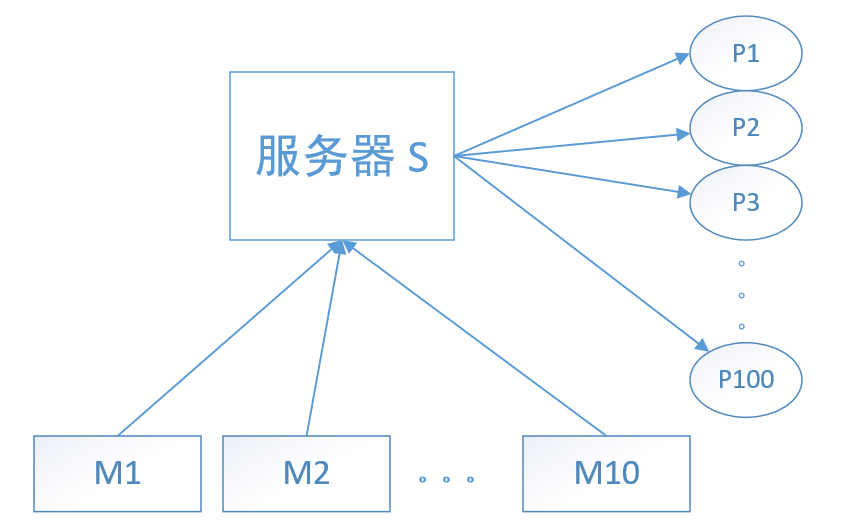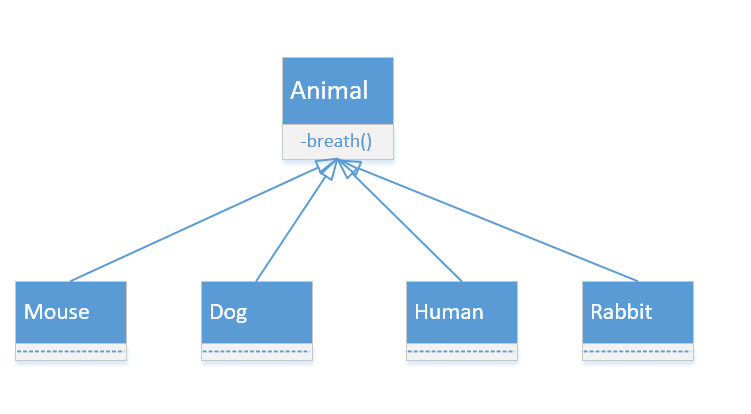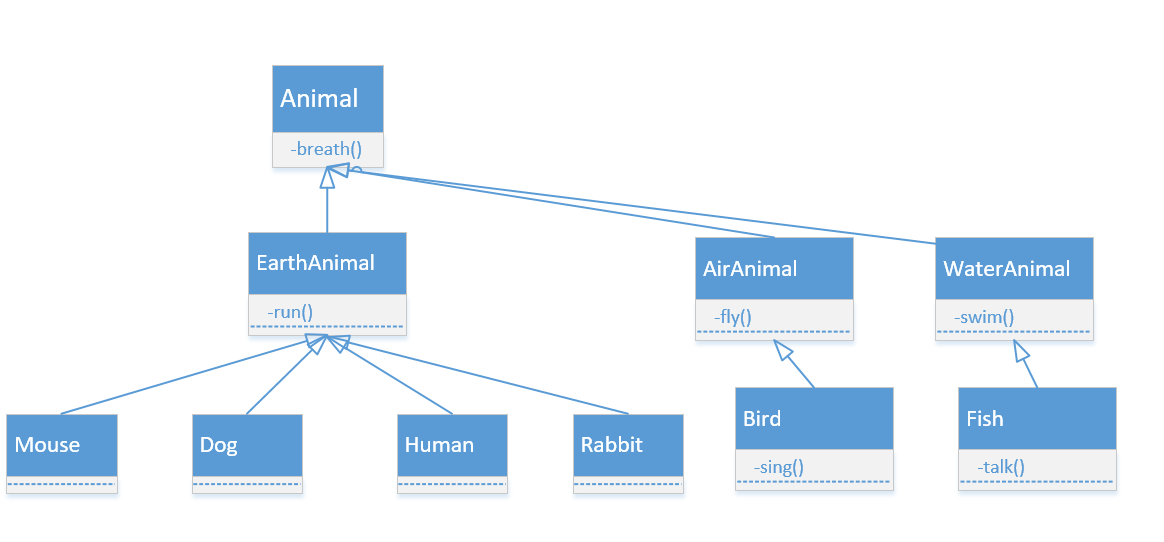# 记录一次面试经历（深刻）

## 面试题目(x1++x100100,y1++y100100,z1++z100100)

## 附（问题的再次解答）

void OnTimer()
{
time.sleep(0.01);
send(x, y, z);
}

typdef stuct Positions {
int x;
int y;
int z;
} Pos;
Queue<Pos> qm;
class Thread
{
\\...
void run{
while(true) {
int x, y, z;
receive(x, y, z);
Pos pp(x,y,z);
qm.enqueue(pp);
}
}
\\...
};

void main()
{
int X;
int Y;
int Z;
while(true) {
time.sleep(0.01);
if(all_data_ready()) {  //判断所有数据是否准备齐全
read_all_data(X, Y, Z);
compute_average(X, Y, Z);
send_all(X, Y, Z);   //发送给100台机器
}
}
}

read_all_data() 的实现是将队列的数据都dequeue，然后放入到X，Y，Z数组中去。